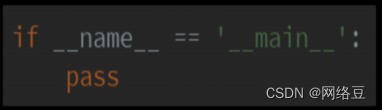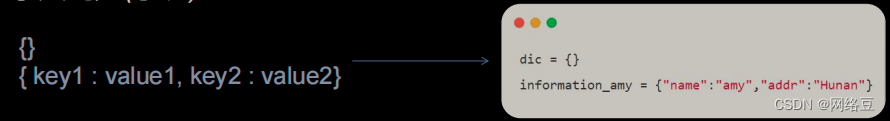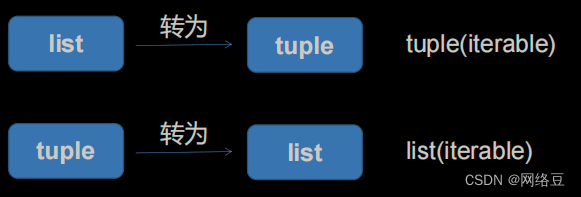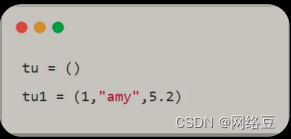# 7款Python数据图表工具的比较

+关注继续查看Python 的科学栈相当成熟，各种应用场景都有相关的模块，包括机器学习和数据分析。数据可视化是发现数据和展示结果的重要一环，只不过过去以来，相对于 R 这样的工具，发展还是落后一些。

1. # Import the pandas library.
2. import pandas
3. Read in the airports data.
5. airports.columns = ["id""name""city""country""code""icao""latitude""longitude""altitude""offset""dst""timezone"
6. Read in the airlines data.
8. airlines.columns = ["id""name""alias""iata""icao""callsign""country""active"
9. Read in the routes data.
11. routes.columns = ["airline""airline_id""source""source_id""dest""dest_id""codeshare""stops""equipment"1. routes = routes[routes["airline_id"] != "\\N"

1. import math
2. def haversine(lon1, lat1, lon2, lat2):
3.     # Convert coordinates to floats.
4.     lon1, lat1, lon2, lat2 = [float(lon1), float(lat1), float(lon2), float(lat2)]
5.     # Convert to radians from degrees.
6.     lon1, lat1, lon2, lat2 = map(math.radians, [lon1, lat1, lon2, lat2])
7.     # Compute distance.
8.     dlon = lon2 - lon1
9.     dlat = lat2 - lat1
10.     a = math.sin(dlat/2)**2 + math.cos(lat1) * math.cos(lat2) * math.sin(dlon/2)**2
11.     c = 2 * math.asin(math.sqrt(a))
12.     km = 6367 * c
13.     return km

1. def calc_dist(row):
2.     dist = 0
3.     try:
4.         # Match source and destination to get coordinates.
5.         source = airports[airports["id"] == row["source_id"]].iloc
6.         dest = airports[airports["id"] == row["dest_id"]].iloc
7.         # Use coordinates to compute distance.
8.         dist = haversine(dest["longitude"], dest["latitude"], source["longitude"], source["latitude"])
9.     except (ValueError, IndexError):
10.         pass
11.     return dist

1. route_lengths = routes.apply(calc_dist, axis=1)

1. import matplotlib.pyplot as plt
2. %matplotlib inline
3.
4. plt.hist(route_lengths, bins=20)1. import seaborn
2.
3. seaborn.distplot(route_lengths, bins=20)1. import numpy
2. # Put relevant columns into a dataframe.
3. route_length_df = pandas.DataFrame({"length": route_lengths, "id": routes["airline_id"]})
4. # Compute the mean route length per airline.
5. airline_route_lengths = route_length_df.groupby("id").aggregate(numpy.mean)
6. # Sort by length so we can make a better chart.
7. airline_route_lengths = airline_route_lengths.sort("length", ascending=False

1. plt.bar(range(airline_route_lengths.shape), airline_route_lengths["length"])Matplotlib的plt.bar方法根据每个数据模型的航空公司平均航线长度(airline_route_lengths[“length”])来做图。

1. def lookup_name(row):
2.     try:
3.         # Match the row id to the id in the airlines dataframe so we can get the name
4.         name = airlines["name"][airlines["id"] == row["id"]].iloc
5.     except (ValueError, IndexError):
6.         name = ""
7.     return name
8. Add the index (the airline ids) as a column
9. airline_route_lengths["id"] = airline_route_lengths.index.copy()
10. # Find all the airline names.
11. airline_route_lengths["name"] = airline_route_lengths.apply(lookup_name, axis=1)
12. # Remove duplicate values in the index
13. airline_route_lengths.index = range(airline_route_lengths.shape)

1. import numpy as np
2. from bokeh.io import output_notebook
3. from bokeh.charts import Bar, show
4. output_notebook()
5. p = Bar(airline_route_lengths, 'name'values='length', title="Average airline route lengths"
6. show(p)

Pygal 是一个能快速制作出有吸引力表格的数据分析库。我们可以用它来按长度分解路由。首先把我们的路由分成短、中、长三个距离，并在 route_lengths 里计算出它们各占的百分比。

1. long_routes = len([k for k in route_lengths if k > 10000]) / len(route_lengths)
2.
3. medium_routes = len([k for k in route_lengths if k < 10000 and k > 2000]) / len(route_lengths)
4.
5. short_routes = len([k for k in route_lengths if k < 2000]) / len(route_lengths)

1. import pygal
2. from IPython.display import SVG
3. chart = pygal.HorizontalBar()
4. chart.title = 'Long, medium, and short routes'
8. chart.render_to_file('routes.svg'
9. SVG(filename='routes.svg'1. name_lengths = airlines["name"].apply(lambda x: len(str(x)))
2.
3. plt.scatter(airlines["id"].astype(int), name_lengths)1. data = pandas.DataFrame({"lengths": name_lengths, "ids": airlines["id"].astype(int)})
2.
3. seaborn.jointplot(x="ids", y="lengths", data=data)1. # Import the basemap package
2. from mpl_toolkits.basemap import Basemap
3. Create a map on which to draw.  We're using a mercator projection, and showing the whole world.
4. m = Basemap(projection='merc',llcrnrlat=-80,urcrnrlat=80,llcrnrlon=-180,urcrnrlon=180,lat_ts=20,resolution='c'
5. # Draw coastlines, and the edges of the map.
6. m.drawcoastlines()
7. m.drawmapboundary()
8. Convert latitude and longitude to x and y coordinates
9. x, y = m(list(airports["longitude"].astype(float)), list(airports["latitude"].astype(float)))
10. # Use matplotlib to draw the points onto the map.
11. m.scatter(x,y,1,marker='o',color='red'
12. # Show the plot.
13. plt.show()

1. import folium
2. # Get a basic world map.
3. airports_map = folium.Map(location=[30, 0], zoom_start=2)
4. # Draw markers on the map.
5. for name, row in airports.iterrows():
6.     # For some reason, this one airport causes issues with the map.
7.     if row["name"] != "South Pole Station"
8.         airports_map.circle_marker(location=[row["latitude"], row["longitude"]], popup=row["name"])
9. Create and show the map.
10. airports_map.create_map('airports.html'
11. airports_map

Folium 使用 leaflet.js 来制作全交互式地图。你可以点击每一个机场在弹出框中看名字。在上边显示一个截屏，但是实际的地图更令人印象深刻。Folium 也允许非常广阔的修改选项来做更好的标注，或者添加更多的东西到地图上。

1. # Make a base map with a mercator projection.  Draw the coastlines.
2. m = Basemap(projection='merc',llcrnrlat=-80,urcrnrlat=80,llcrnrlon=-180,urcrnrlon=180,lat_ts=20,resolution='c'
3. m.drawcoastlines()
4. # Iterate through the first 3000 rows
5. for name, row in routes[:3000].iterrows():
6.     try:
7.         # Get the source and dest airports.
8.         source = airports[airports["id"] == row["source_id"]].iloc
9.         dest = airports[airports["id"] == row["dest_id"]].iloc
10.         # Don't draw overly long routes.
11.         if abs(float(source["longitude"]) - float(dest["longitude"])) < 90:
12.             # Draw a great circle between source and dest airports.
13.             m.drawgreatcircle(float(source["longitude"]), float(source["latitude"]), float(dest["longitude"]), float(dest["latitude"]),linewidth=1,color='b'
14.     except (ValueError, IndexError):
15.         pass
16.
17. # Show the map.
18. plt.show()1. # Initialize the weights dictionary.
2. weights = {}
3. # Keep track of keys that have been added once -- we only want edges with a weight of more than 1 to keep our network size manageable.
5. # Iterate through each route.
6. for name, row in routes.iterrows():
7.     # Extract the source and dest airport ids.
8.     source = row["source_id"
9.     dest = row["dest_id"
10.
11.     # Create a key for the weights dictionary.
12.     # This corresponds to one edge, and has the start and end of the route.
13.     key = "{0}_{1}".format(source, dest)
14.     # If the key is already in weights, increment the weight.
15.     if key in weights:
16.         weights[key] += 1
17.     # If the key is in added keys, initialize the key in the weights dictionary, with a weight of 2.
19.         weights[key] = 2
20.     # If the key isn't in added_keys yet, append it.
21.     # This ensures that we aren't adding edges with a weight of 1.
22.     else

1. # Import networkx and initialize the graph.
2. import networkx as nx
3. graph = nx.Graph()
4. # Keep track of added nodes in this set so we don't add twice.
5. nodes = set()
6. # Iterate through each edge.
7. for k, weight in weights.items():
8.     try:
9.         # Split the source and dest ids and convert to integers.
10.         source, dest = k.split("_"
11.         source, dest = [int(source), int(dest)]
12.         # Add the source if it isn't in the nodes.
13.         if source not in nodes:
15.         # Add the dest if it isn't in the nodes.
16.         if dest not in nodes:
18.         # Add both source and dest to the nodes set
19.         # Sets don't allow duplicates.
22.
23.         # Add the edge to the graph.
25.     except (ValueError, IndexError):
26.         pass
27. pos=nx.spring_layout(graph)
28. # Draw the nodes and edges.
29. nx.draw_networkx_nodes(graph,pos, node_color='red', node_size=10, alpha=0.8)
30. nx.draw_networkx_edges(graph,pos,width=1.0,alpha=1)
31. # Show the plot.
32. plt.show()7 款 Python 数据图表工具的比较
1759 0Python编程 条件判断语句
Python编程 条件判断语句
15 0Python编程 顺序执行与程序的主入口
Python编程 顺序执行与程序的主入口
11 0Python编程 集合
Python编程 集合
13 0Python编程 字典的常用操作
Python编程 字典的常用操作
10 0Python编程 字典创建map与Zip
Python编程 字典创建map与Zip
12 0Python编程 字典创建
Python编程 字典创建
15 0Python编程 元组中不允许的操作
Python编程 元组中不允许的操作
11 0Python编程 元组的创建
Python编程 元组的创建
9 0

Python基础快速入门实战教程378008